Disclosure: This post may contain affiliate links, meaning when you click the links and make a purchase, we receive a commission.

# How to Compare Two Columns or Lists in Excel

While doing different tasks in Excel we often come across a situation where the matching and differences of two or multiple columns are required. It’s not a difficult task to compare two lists in excel but you may get confused as there are so many ways to do it. In this article, we will look for different techniques of matching and differentiating columns in Excel. First of all, we will be using a table of 2 columns containing different colors’ names.

#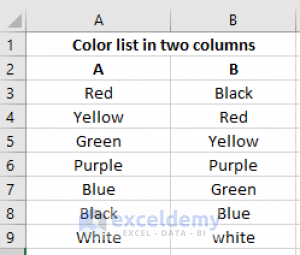## Compare two columns in excel and highlight differences

Using conditional formatting is a way of finding the differences between two columns in Excel. The procedure is given below.

• At first, select the data range that you wish to highlight from two columns.
• Then click on to New Rule from Conditional Formatting option from your Home tab• Click Use a formula to determine which cells to format under the Select a Rule Type list box.
• Enter this formula: =\$A3<>\$B3 into the Format values where this formula is true text box and then press on to the Format option.• A new dialogue box of Format Cells will come up, please specify a color of your choice for highlighting the differences under the Fill tab.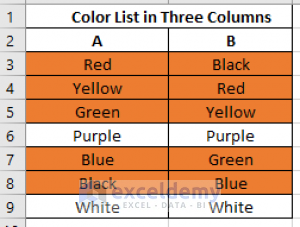## Compare two lists in excel and highlight matches

### 1)  Using the Match function

IF function plays a significant role in finding both differences and matches in excel. Here we will be using it to find the matches in two columns.

• As you can see there is a match in cell A6 and B6. Let’s select the cell C6 for applying the formula.
• `Then use the formula =IF(A3=B3,"Match","")`

In the cell C2, there is no match so nothing will appear if we drag down the cell where the two rows match there we will found our result.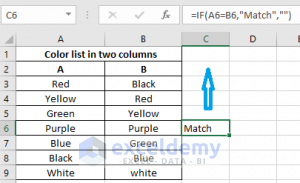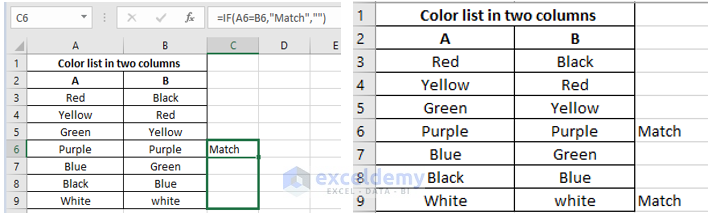### 2) Using conditional formatting

Again, conditional formatting can be used for finding the matches between two columns in Excel. The procedure is given below.

• First, select the data range that you wish to highlight from two columns.
• Then click on to New Rule from the Conditional Formatting option from your Home tab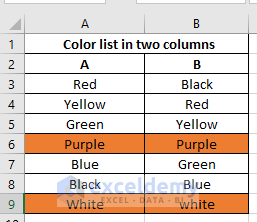## Excel formula to compare two columns and return a value

### 1) Using IF function for determining differences in two columns

• Use a vacant cell that is in same rows with A3 and B3. Let`s choose C3 cell here.
• `Then put the formula =IF(A3<>B3,"No match","") `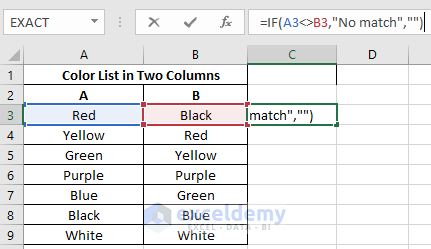• By dragging down the box where the formula was applied (in C3) in the specified column the result of the two columns will be found. By doing the dragging the formula will be applied to the other cells.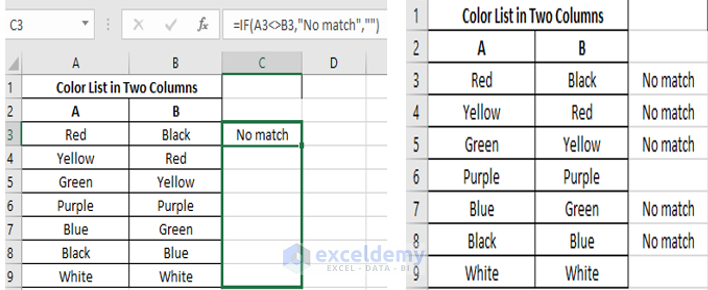### 2) Using Exact Function

To show the differences between two column the easiest option is using the Exact option. It has to be remembered that it can be used for only two columns and this function is case sensitive. The procedure of using Exact function is given below.

• Firstly, choose the column where the result has to be shown. In our worksheet, Column A and B shows two color list. The color is shown from A3 and B3 respectively. In A2 and B2 the headings are A and B. Let’s say we want to show our result in Column C.
• Click on C3.
• Click on the Formulas tab on the Ribbon and then on the Text option.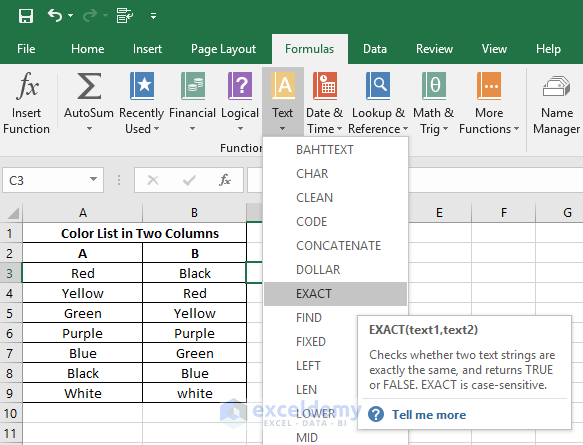• Under Text option, click on the formula titled Exact.
• Under the Excel’s Function Arguments window write A3 on Text1 and B3 on Text2.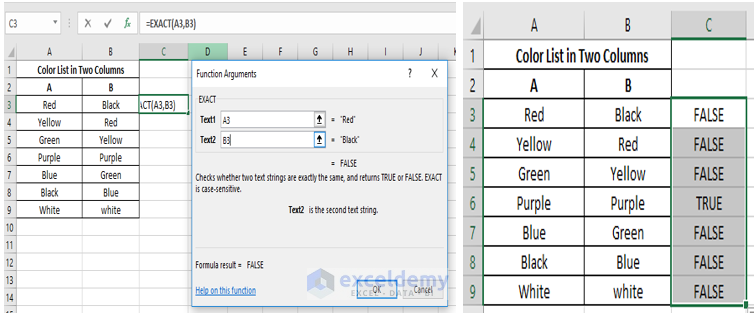• The matched and unmatched result will be shown on C3.
• By dragging the formulated cell C3 on downwards the whole column result will be found.

Note that this function is case sensitive. If it was not case sensitive we would have got True in C9.

### 3) Compare two tables in Excel using VLOOKUP and IF

If you are having 2 tables of different and exact data in their column and we need to find the matches and differences you can use a formula
=IF(VLOOKUP(Value, Table-Reference, Index-number, False)=Value,”Match”, “Do Not Match”).

Here if the amount of 1st record=amount of 2nd record and invoice of 1st record= invoice of 2nd record then statement Match will return in the cell otherwise it will return Do Not Match. The procedure is given below.

• Indicate a vacant cell where we want to find the matches and differences of 1st rows of the two cells.
• Let’s say we want to choose Column D.
• `In D3 put,`
`=IF(VLOOKUP(A3,G3:H9,2,FALSE)=B3,"Match","Do Not Match")`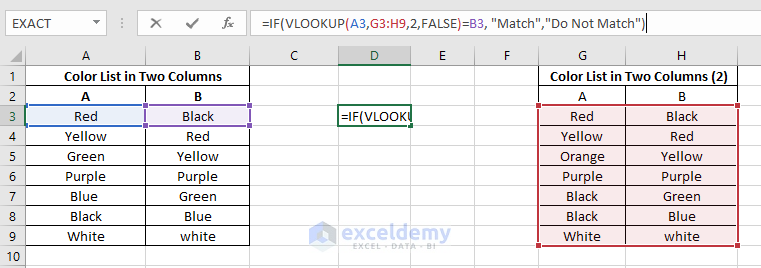• After pressing enter we will see that the result ‘Match’ is shown. This is because the 1st row of both the tables contains the same variables.
• By dragging the cell D3 in downwards we will find the result for the rest.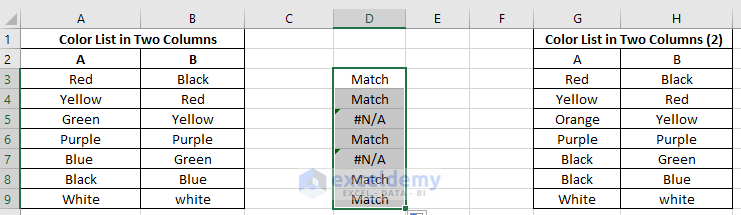Here one thing is to be noted that we are doing the analysis of cells containing text. That’s why the result Do Not Match is not shown. If we use a number instead of text, we will see the result Do Not Match in the column D. Let`s use number instead of text in the rows of two tables and see the result.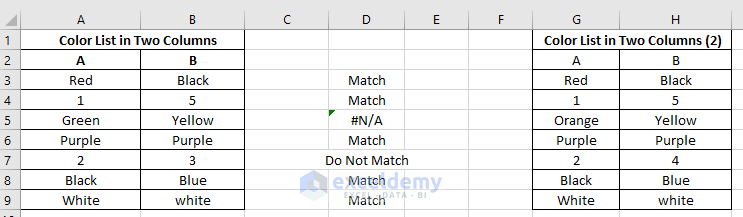# How to Compare Two Columns in Excel Using VLOOKUP

### 4) Using IF function to determine Matches and Differences in separate Column

Previously we discussed how the differences and matches are found using IF function. Now we will be using the IF function to do both. The formula we will be using is

`=IF(A3=B3,"Match","No match") Or`
`=IF(A3<>B3,"No match", "Match") `

in a different empty column (here we used C3). By dragging the cell downwards where the formula is applied we can find the matching and differences between the two columns.## How to compare two columns in different excel sheets

### 1) For finding differences

Most of us don’t know that it is possible to compare data from 2 different worksheets/spreadsheets. By using conditional formatting, it can be easily done. For that, we have to use a formula of =A3<>Sheet2!A3.

• After opening two spreadsheets, let’s say we want to find the differences in spreadsheets 1 with spreadsheets 2.
• In your Home tab, in the Styles group, let`s click New rule from Conditional Formatting option, and create a rule with the formula =A3<>Sheet2!A3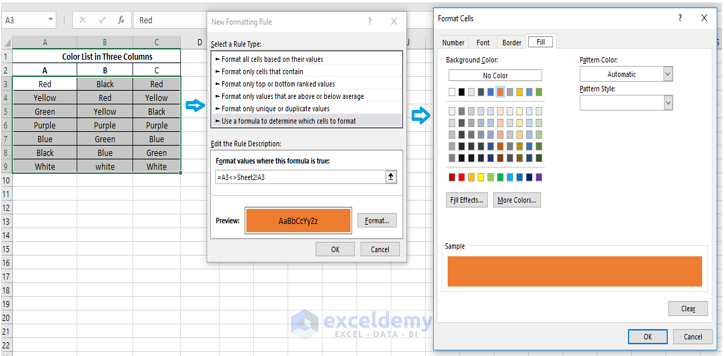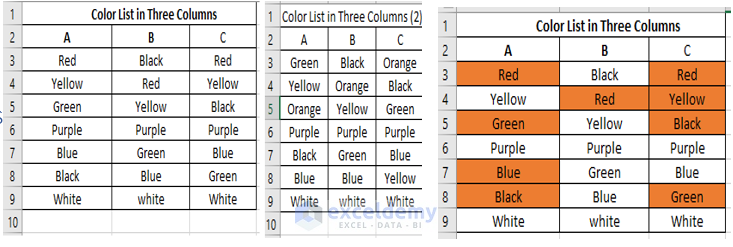### 2) For finding matches

Just like finding the differences between two worksheets/spreadsheets, you can also find the matches in the same manner. Only the formula will be changed. Here we will be using the formula
=(A3=Sheet2!A3) to find out the matches.

• After opening two spreadsheets, let’s say we want to find the differences in spreadsheets 1 with spreadsheets 2.
• In your Home tab, in the Styles group, let`s click New rule from Conditional Formatting option, and create a rule with the formula =(A3=Sheet2!A3)## Comparing multiple columns in excel

Here we will deal with 3 columns.

#### 1) Using AND or COUNTIF function for finding matches

For highlighting rows that have same/identical variables in every column you can create conditional formatting rules based on the formula =AND(\$A3=\$B3, \$A3=\$C3) or =COUNTIF(\$A3:\$C3, \$A3)=3 ,where the comparing column number is 3 and the cells A2, B2, and C2 are the top cells.Click on the image to get a full view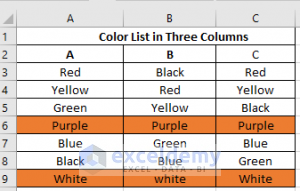### 2) Using IF and AND functions for finding differences

Just like 2 columns finding differences in multiple columns is also easy. The procedure is given below.

• Let`s choose a vacant cell say C3.
• `Then put the formula,`
`Source code=IF(AND(A3<>B3, B3<>C3), "No Match", "Match")`
• Again, by dragging down the box, the formula will be applied to the other cells.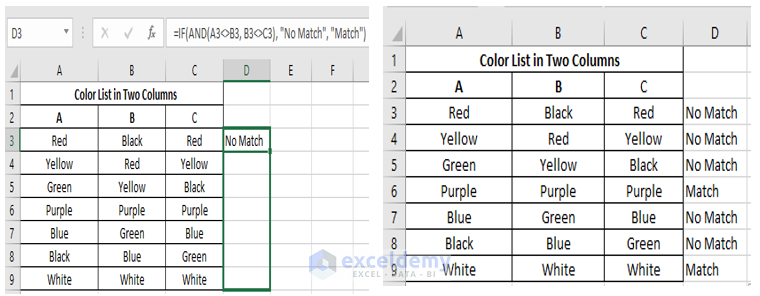## Conclusion

While doing different data analysis we face difficulties of finding differences and matches in columns in Excel. As you can see there are a lot of ways in Excel to find them. Based on the task you are doing you can use any of these. This Blog may help you to overcome the problems that you face while working on multiple columns.

Hello!

Welcome to my Profile. Here I will be posting articles related to Microsoft Excel. I have completed my BSc in Electrical and Electronic Engineering from American International University-Bangladesh. I am a diligent, goal-oriented engineer with an immense thirst for knowledge and attitude to grow continuously. Continuous improvement and life-long learning is my motto.

1. ReplyFarhana Alam Feb 19, 2018 at 10:16 PM

Very informative and well described.

• ReplyFeb 20, 2018 at 5:25 PM

Thanks, Farhana for the feedback.

2. ReplySteve Feb 21, 2018 at 8:53 PM

Excellent! Thanks for this useful information.

• ReplyFeb 22, 2018 at 11:53 AM

Thanks. Glad to know that you found it useful.